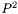#Boost C++ Libraries

...one of the most highly regarded and expertly designed C++ library projects in the world.

This is the documentation for an old version of boost. Click here for the latest Boost documentation.

## Struct template weighted_p_square_quantile_impl

boost::accumulators::impl::weighted_p_square_quantile_impl — Single quantile estimation with thealgorithm for weighted samples.

## Synopsis

```// In header: <boost/accumulators/statistics/weighted_p_square_quantile.hpp>

template<typename Sample, typename Weight, typename Impl>
struct weighted_p_square_quantile_impl {
// types
typedef numeric::functional::multiplies< Sample, Weight >::result_type            weighted_sample;
typedef numeric::functional::average< weighted_sample, std::size_t >::result_type float_type;
typedef array< float_type, 5 >                                                    array_type;
typedef float_type                                                                result_type;

// construct/copy/destruct
template<typename Args> weighted_p_square_quantile_impl(Args const &);

// public member functions
template<typename Args> void operator()(Args const &) ;
result_type result(dont_care) const;
};```

## Description

This version of thealgorithm extends thealgorithm to support weighted samples. Thealgorithm estimates a quantile dynamically without storing samples. Instead of storing the whole sample cumulative distribution, only five points (markers) are stored. The heights of these markers are the minimum and the maximum of the samples and the current estimates of the-,- and-quantiles. Their positions are equal to the number of samples that are smaller or equal to the markers. Each time a new sample is added, the positions of the markers are updated and if necessary their heights are adjusted using a piecewise- parabolic formula.

For further details, see

R. Jain and I. Chlamtac, The P^2 algorithmus for dynamic calculation of quantiles and histograms without storing observations, Communications of the ACM, Volume 28 (October), Number 10, 1985, p. 1076-1085.

### `weighted_p_square_quantile_impl` public construct/copy/destruct

1. `template<typename Args> weighted_p_square_quantile_impl(Args const & args);`

### `weighted_p_square_quantile_impl` public member functions

1. `template<typename Args> void operator()(Args const & args) ;`
2. `result_type result(dont_care) const;`